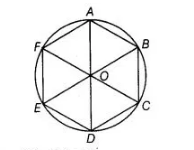# If a hexagon ABCDEF circumscribe a circle,

Question:

If a hexagon ABCDEF circumscribe a circle, prove that

AB + CD + EF =BC + DE + FA

Solution:

Given A hexagon ABCDEF circumscribe a circle.To prove $A B+C D+E F=B C+D E+F A$

Proof $\quad A B+C D+E F=(A Q+Q B)+(C S+S D)+(E U+U F)$

$=A P+B R+C R+D T+E T+F P$

$=(A P+F P)+(B R+C R)+(D T+E T)$

$A B+C D+E F=A F+B C+D E$

$A Q=A P$

$Q B=B R$

$C S=C R$

$D S=D T$

$E U=E T$

[tangents drawn from an external point to a circle are equal]

Hence proved.